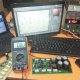Breaking News
Home / making transformer / Wire gauge for transformer

# Wire gauge for transformer:

Today we are going to learn that how to make a transformer. and also we can learn which gauge of wire we need for our transformer. at first, we have to decide where we are going to use the transformer? Such as Charger, amplifier, DC fan, Light. for our need, we will decide wire gauge for the transformer.

## For an example: Amplifier

Let’s go to make a transformer for an amplifier.

We want to make the 1000Watts amplifier.  for 1000Watts we need a 1000Watts transformer first. let’s go to make a 1000Watts transformer. if you don’t know  that how to make transformer read this article first,  Transformer,

For Primary Binding: our primary voltage is 220v AC. We know that Watts/Voltage=Amperes. So, 1000/220=4.5. our primary ampere will be 4.5. so we have to use 4.5-ampere wire. Let’s go to know which wire can give 4.5 amperes.

Gauge     –      Ampere

7              –        44.2

8              –        33.3

9              –        26.5

10            –        21.2

11            –        16.6

12            –        13.5

13            –        10.5

14            –          8.3

15            –          6.6

16            –          5.2

17            –          4.1

18            –          3.2

19            –          2.6

20            –          2.0

21            –          1.6

22            –          1.2

23            –          1.0

24            –          0.8

25            –          0.6

26            –          0.5

27            –          0.4

28            –          0.3

29            –          0.29

30            –          0.22

So our wire will be 16 or 17 as you want. number 16 is for 5.2 amperes and number 17 is for 4.1 amperes.

Now we are going to select the Secondary wire. our amplifier is 1000 watts. We know that voltage X ampere= Watts. our voltage will be 50. so Watts / Voltage = Ampere. So, 1000/50=20. our secondary ampere will be 20 amperes. We can see in the ampere chart that Gauge number 10 is for 20 amperes. so we have to use 10 gauge number wire.

let’s watch a video

transistor circuit diagram of 2sa1943 and 2sc5200

Thanks a lot to be with us. If you want another post then please visit our website.

We have another post for you. like as repairing amplifier,